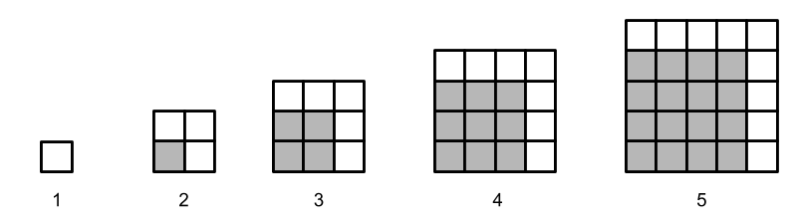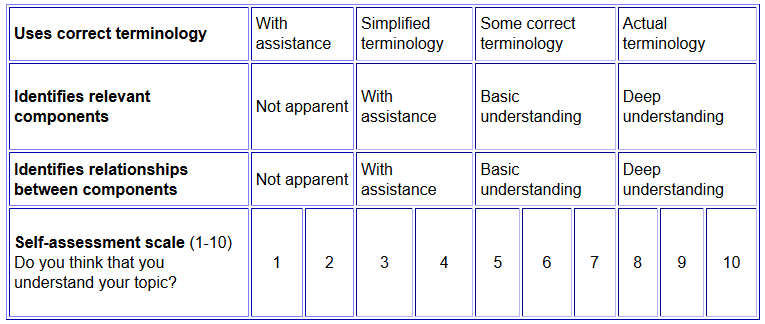# SILO 2.1 (DRAFT)

## Year 2, Term 1: Algebra and algorithms

### Learning intention: Students explore number patterns as an introduction to algebra and algorithms.

NSW Syllabus
Australian Curriculum (version 9.0)
"A student describes, follows and represents algorithms to solve problems." (ST1-3DP-T)
"Students learn to recognise, describe and create additive patterns that increase or decrease by a constant amount, using numbers, shapes and objects, and identify missing elements in the pattern." (AC9M2A01)

## Week 1

Number formula activity

• Students choose a number between 1 and 100 and write it in a box in the middle of a sheet of paper (e.g., "14")
• Students write as many equations for this number as they can fit on the page (e.g., 10 + 4; 2 x 7, 20 - 6, 10+1+1+2, and so on).

Number guessing game

• The teacher chooses a secret number between 1 and 100 and writes it on the board before covering it over to conceal the number.
• Students have 7 guesses to discover the number.
• The teacher can only give "Yes" or "No" answers.
• Reminders are written on the board to narrow down the options (e.g., if a students asked "Is it odd?" and the answer was "No", the teacher would write "Even" on the board.
• Guesses are tallied up on the board and the answer is revealed after the seventh guess unless guessed earlier.

The game is played again with a student running the game.

The next stage with this activity is to contrast good guesses such as "Is it lower than 50?" with random guesses such as "Is it 87?"  This can then be used as an introduction to algorithms where the aim is to narrow down the options.

Introduction to algebra

What's in the black box?

The following video (8:59) provides another introduction to algebra using boxes.

## Week 2

Geometric patterns## Week 3

Algorithms in other curriculum areas

The following video (2:09) shows how algorithms are also used in other curriculum areas.

## Week 4

Algorithms and pseudo code

The following video (4:58) explains algorithms using a counting example and pseudo code.

## Week 10

Moderated self-assessment